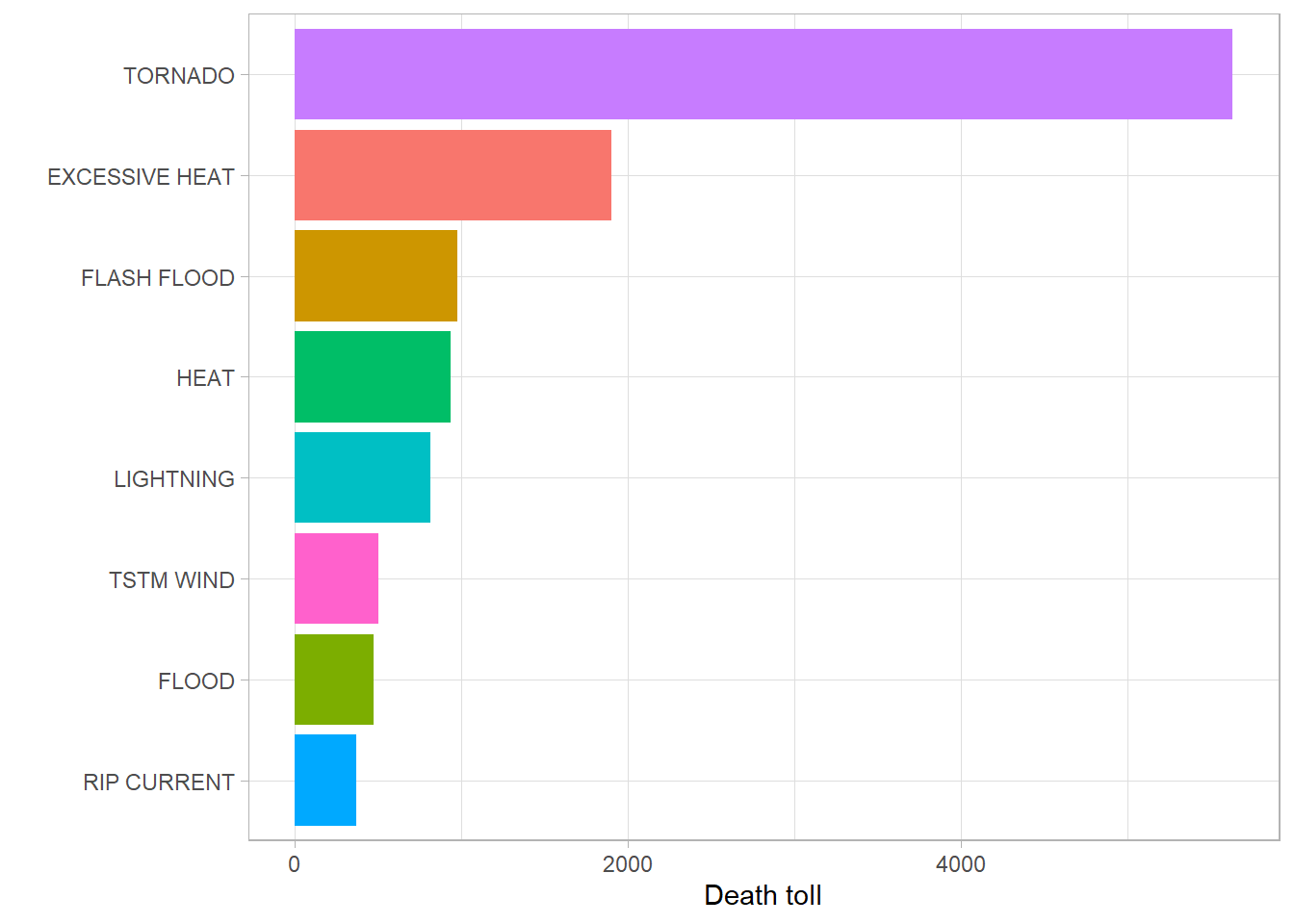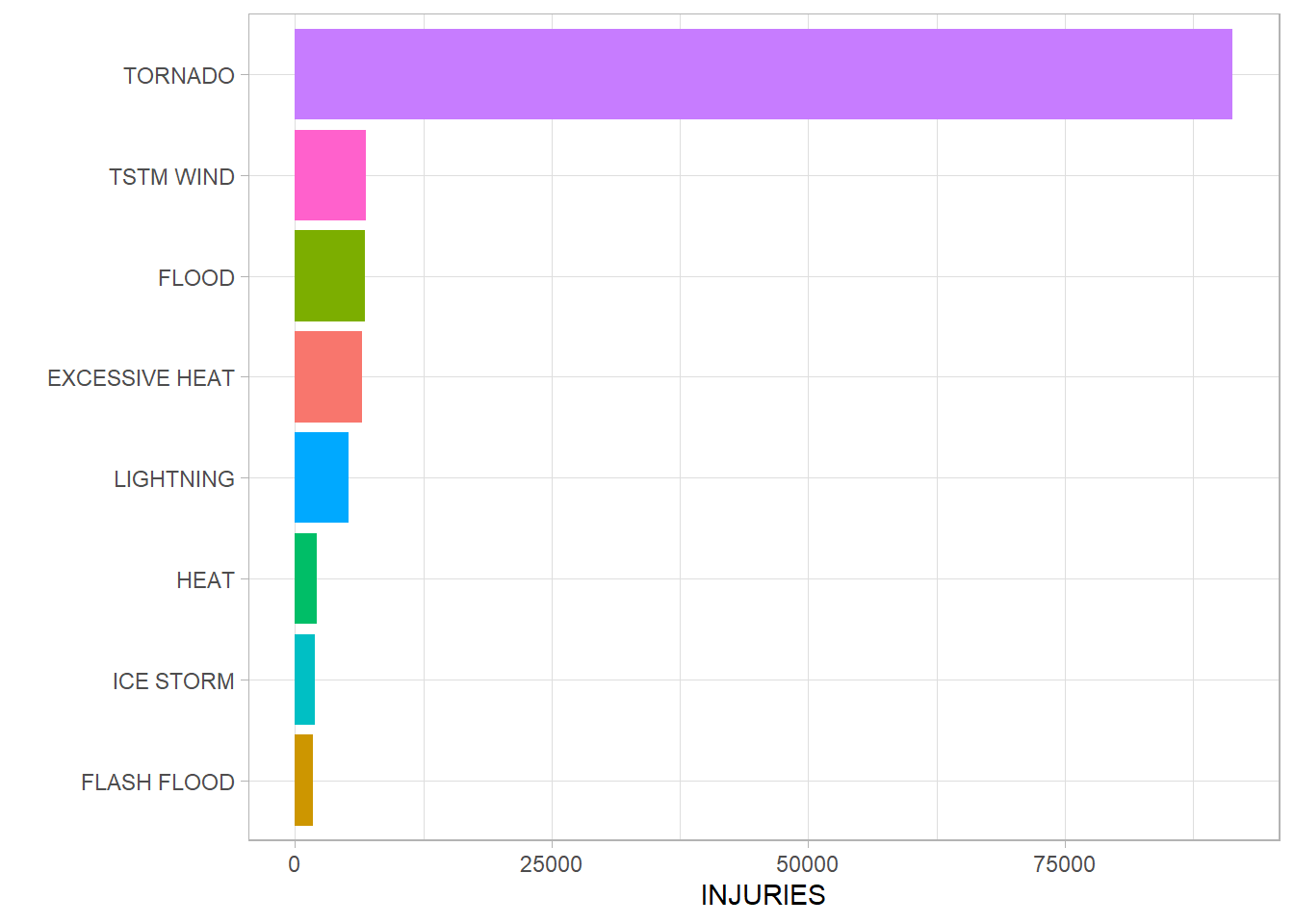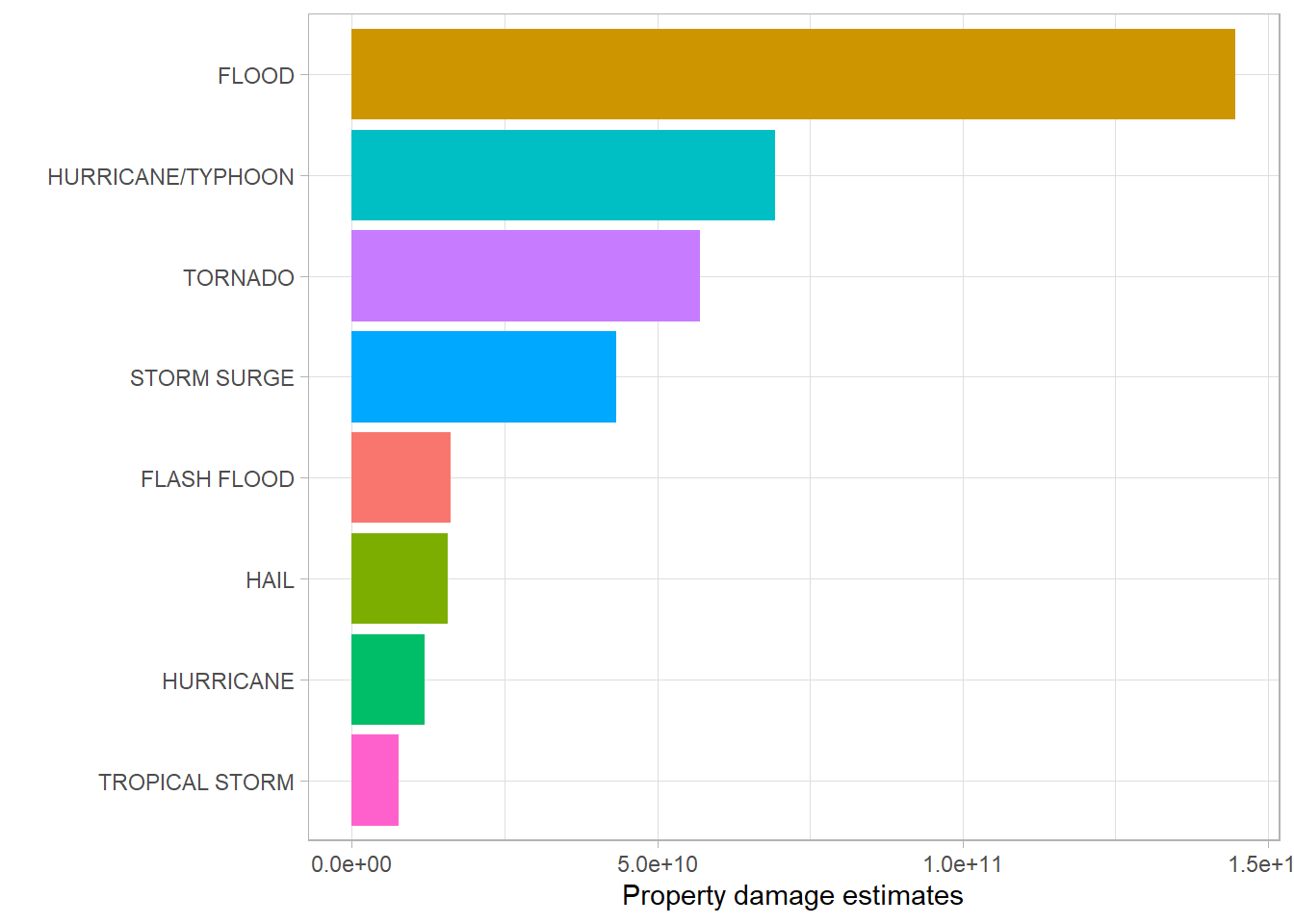##Sypnosis
Weather events have a huge impact on public health and economic problems. In this analysis, we will look which types of events leads to the most severe impacts with the data we get from The U.S. National Oceanic and Atmospheric Administration’s (NOAA).

##Data Processing
We will use dataset from The U.S. National Oceanic and Amospheric Administration’s(NOAA) storm database.
Libraries we’ll use are:

download.file(url = "https://d396qusza40orc.cloudfront.net/repdata%2Fdata%2FStormData.csv.bz2", destfile = "StormData")

StormData <- read.csv(("StormData"),sep = ",",header=TRUE)

Estimate property damages are in dollars, 3 digits follwed by an alphabet, “K” for “1000”, “M/m” for “million”, “B” for “billion”. So, we have to change these into numerical values in the dataset.

table(StormData$PROPDMGEXP) ## ## - ? + 0 1 2 3 4 5 6 ## 465934 1 8 5 216 25 13 4 4 28 4 ## 7 8 B h H K m M ## 5 1 40 1 6 424665 7 11330 StormData$PROPDMGEXP2 <- 1
StormData$PROPDMGEXP2[which(StormData$PROPDMGEXP == "K")] <- 1000
StormData$PROPDMGEXP2[which(StormData$PROPDMGEXP == "M" | StormData$PROPDMGEXP == "m")] <- 1000000 StormData$PROPDMGEXP2[which(StormData$PROPDMGEXP == "B")] <- 1000000000 table(StormData$PRODMGEXP2)
## < table of extent 0 >

#Question 1: Which types of events are most harmfult to population health? Fatalities and injuries have the most impact on public health, so we can use 2 plots to see which types of events have the most impact on public health.

The first plot will present Fatalities by Event Type.

StormData %>%
select(FATALITIES, EVTYPE) %>%
group_by(EVTYPE) %>%
summarise(SumFATALITIES = sum(FATALITIES)) %>%
top_n(n = 8, wt = SumFATALITIES) %>%
ggplot(aes(y = SumFATALITIES, x = reorder(x = EVTYPE, X = SumFATALITIES), fill=EVTYPE))+
geom_bar(stat = "identity", show.legend = FALSE) +
#geom_text(aes(label=SumFATALITIES), size = 4, hjust = 0.5, vjust = -0.1) +
xlab(label = "") +
ylab(label = "Death toll") +
coord_flip() +
theme_light()
## summarise() ungrouping output (override with .groups argument)The second plot presents Injuries by Event Type.

StormData %>%
select(INJURIES, EVTYPE) %>%
group_by(EVTYPE) %>%
summarise(SumINJURIES = sum(INJURIES)) %>%
top_n(n = 8, wt = SumINJURIES) %>%
ggplot(aes(y = SumINJURIES, x = reorder(x = EVTYPE, X = SumINJURIES), fill=EVTYPE))+
geom_bar(stat = "identity", show.legend = FALSE) +
#geom_text(aes(label=SumINJURIES), size = 4, hjust = 0.5, vjust = -0.1) +
xlab(label = "") +
ylab(label = "INJURIES") +
coord_flip() +
theme_light()
## summarise() ungrouping output (override with .groups argument)#Question 2: Which type of events have the most impact on economic consequences?

This plot presents Property Damage Estimates by Event Type.

StormData %>%
select(PROPDMG, PROPDMGEXP2, EVTYPE) %>%
group_by(EVTYPE) %>%
mutate(SumPROPDMGEXP = (PROPDMG * PROPDMGEXP2)) %>%
summarise(SumPROPDMGEXP2 = sum(SumPROPDMGEXP)) %>%
top_n(n = 8, wt = SumPROPDMGEXP2) %>%
ggplot(aes(y = SumPROPDMGEXP2, x = reorder(x = EVTYPE, X = SumPROPDMGEXP2), fill=EVTYPE))+
geom_bar(stat = "identity", show.legend = FALSE) +
#geom_text(aes(label=SumFATALITIES), size = 4, hjust = 0.5, vjust = -0.1) +
xlab(label = "") +
ylab(label = "Property damage estimates") +
coord_flip() +
theme_light()
## summarise() ungrouping output (override with .groups argument)#Results
Tornado have the most impact of public health in both fatalities and injuries.
Flood have the most impact on economic consequenses.